# Body Fluid Compartments Part 1 Body Composition E

• Slides: 19Body Fluid Compartments – Part 1 Body Composition E. S. Prakash, Faculty of Medicine, AIMST University E-mail: [email protected] com Note to Readers – This is one of the lectures in the Molecular & Cellular Basis of Medicine course, Element 2 [General Physiology, Nerve – Muscle Physiology, Basics of Hematology] in Term 1 of Year 1 of the MBBS programme.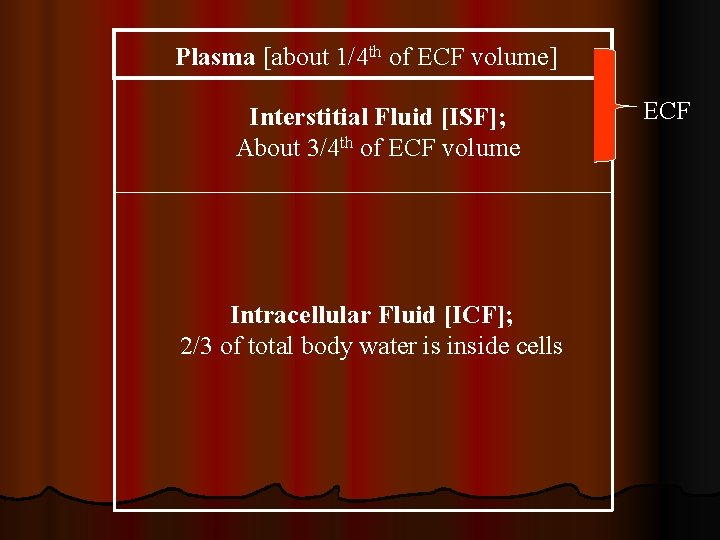Plasma [about 1/4 th of ECF volume] Interstitial Fluid [ISF]; About 3/4 th of ECF volume Intracellular Fluid [ICF]; 2/3 of total body water is inside cells ECF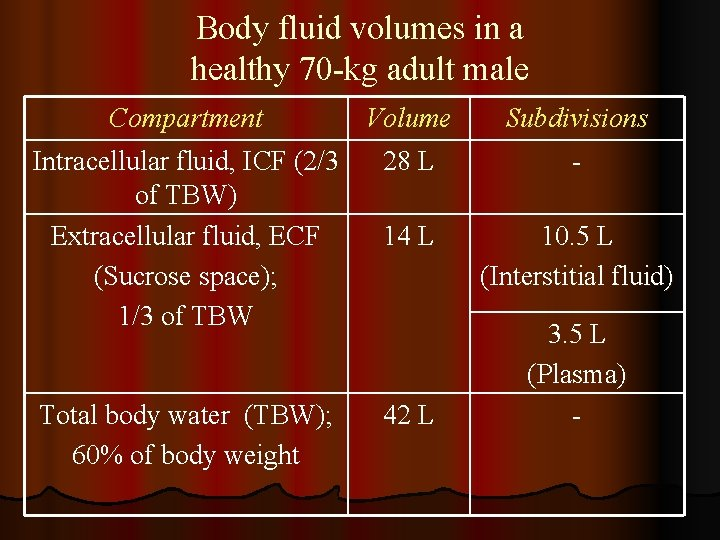Body fluid volumes in a healthy 70 -kg adult male Compartment Volume Subdivisions Intracellular fluid, ICF (2/3 of TBW) Extracellular fluid, ECF (Sucrose space); 1/3 of TBW 28 L - 14 L 10. 5 L (Interstitial fluid) Total body water (TBW); 60% of body weight 42 L 3. 5 L (Plasma) -Measuring body fluid volumes: l Principle: Indicator-dilution principle l Method: 1. Administer a known amount (A) of a substance that will get distributed in a particular body fluid compartment; 2. Determine the final steady state concentration (C) of this indicator in blood; 3. Volume of distribution Vd of the indicator = Amount of indicator injected (A) Concentration in blood after mixing (C)Indicators used for measuring plasma volume, ECF volume and total body water Compartment Criterion Plasma ECF volume Total body water (TBW) Substance should not cross capillaries Indicators Evans blue dye; radioiodinated fibrinogen; radioiodinated albumin Substance should Isotonic solutions of sucrose, cross capillaries inulin, mannitol, Na. Cl but not cross cell membranes Substance Heavy water, tritiated water, distributes evenly aminopyrine, antipyrine in ICF & ECFTake this problem: l A. B. C. D. 100 mg of sucrose is injected into a 70 kg man. The plasma sucrose level after mixing is 0. 01 mg/ml. If 5 mg has been metabolized during this period, then, what is the ECF volume? 9. 5 L 14 L 17. 5 L 10 L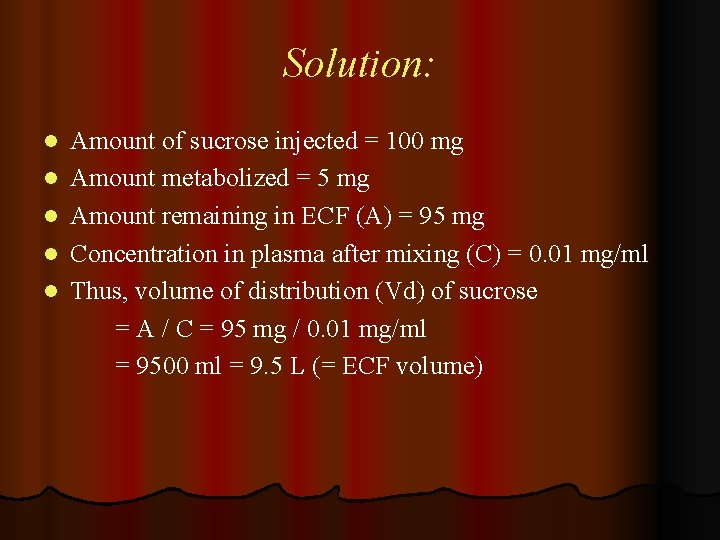Solution: l l l Amount of sucrose injected = 100 mg Amount metabolized = 5 mg Amount remaining in ECF (A) = 95 mg Concentration in plasma after mixing (C) = 0. 01 mg/ml Thus, volume of distribution (Vd) of sucrose = A / C = 95 mg / 0. 01 mg/ml = 9500 ml = 9. 5 L (= ECF volume)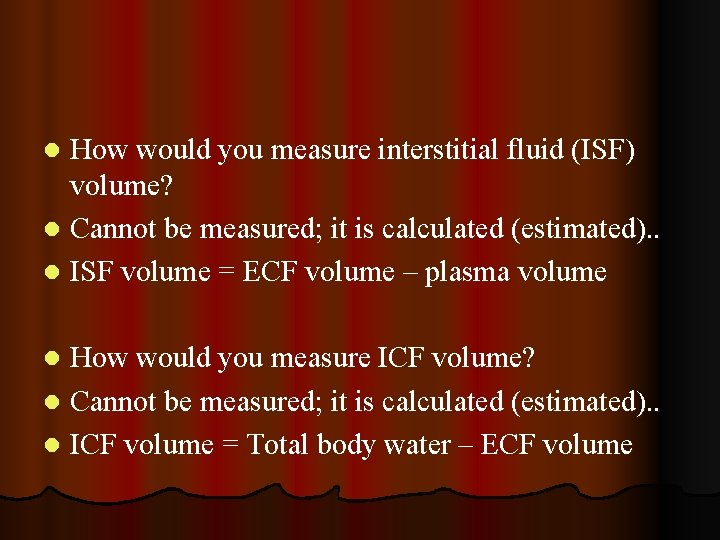How would you measure interstitial fluid (ISF) volume? l Cannot be measured; it is calculated (estimated). . l ISF volume = ECF volume – plasma volume l How would you measure ICF volume? l Cannot be measured; it is calculated (estimated). . l ICF volume = Total body water – ECF volume l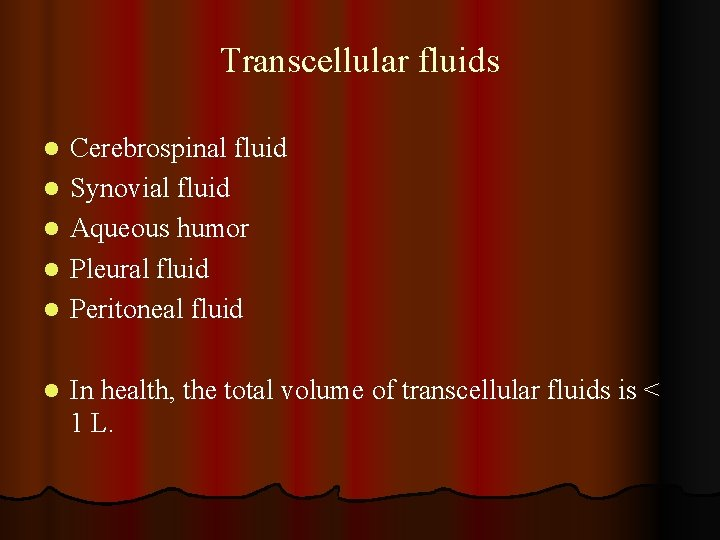Transcellular fluids l l l Cerebrospinal fluid Synovial fluid Aqueous humor Pleural fluid Peritoneal fluid In health, the total volume of transcellular fluids is < 1 L.What is the relationship between blood volume and plasma volume? l Blood = plasma + cells in blood l Volume of cells: packed cell volume (PCV) l PCV is also called hematocrit (Hct) l Blood volume = plasma volume × 100 / (100 – Hct) Image from http: //users. rcn. com/jkimball. ma. ultranet/Biology. Pages /B/Blood. html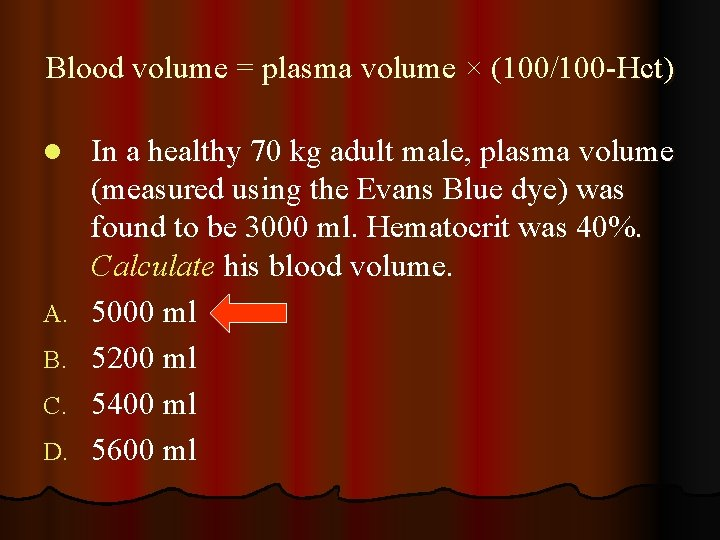Blood volume = plasma volume × (100/100 -Hct) l A. B. C. D. In a healthy 70 kg adult male, plasma volume (measured using the Evans Blue dye) was found to be 3000 ml. Hematocrit was 40%. Calculate his blood volume. 5000 ml 5200 ml 5400 ml 5600 ml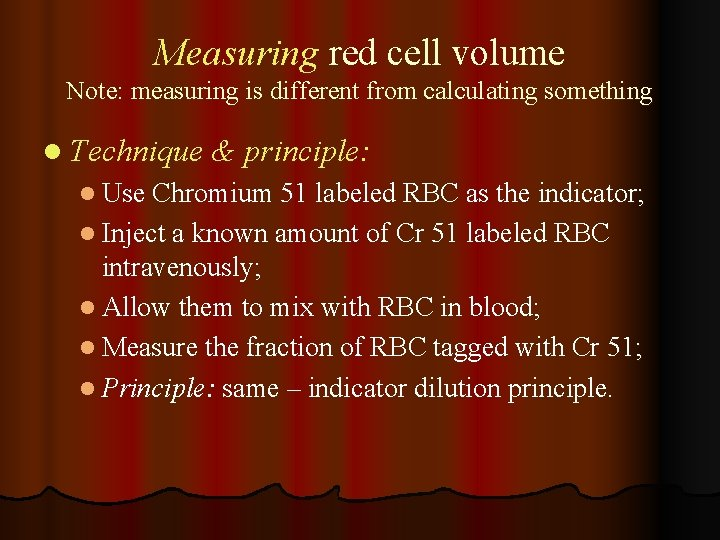Measuring red cell volume Note: measuring is different from calculating something l Technique & principle: l Use Chromium 51 labeled RBC as the indicator; l Inject a known amount of Cr 51 labeled RBC intravenously; l Allow them to mix with RBC in blood; l Measure the fraction of RBC tagged with Cr 51; l Principle: same – indicator dilution principle.Lean body mass (LBM) l l l Definition: LBM is fat free mass Total body mass = fat mass + fat free mass Note: fat is relatively anhydrous Note: the water content of LBM is constant Water content of LBM is constant - 70 ml /100 g tissue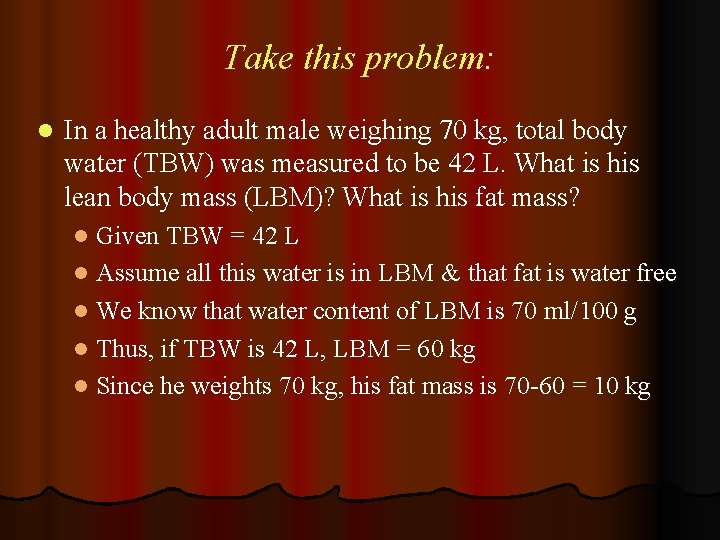Take this problem: l In a healthy adult male weighing 70 kg, total body water (TBW) was measured to be 42 L. What is his lean body mass (LBM)? What is his fat mass? l Given TBW = 42 L l Assume all this water is in LBM & that fat is water free l We know that water content of LBM is 70 ml/100 g l Thus, if TBW is 42 L, LBM = 60 kg l Since he weights 70 kg, his fat mass is 70 -60 = 10 kg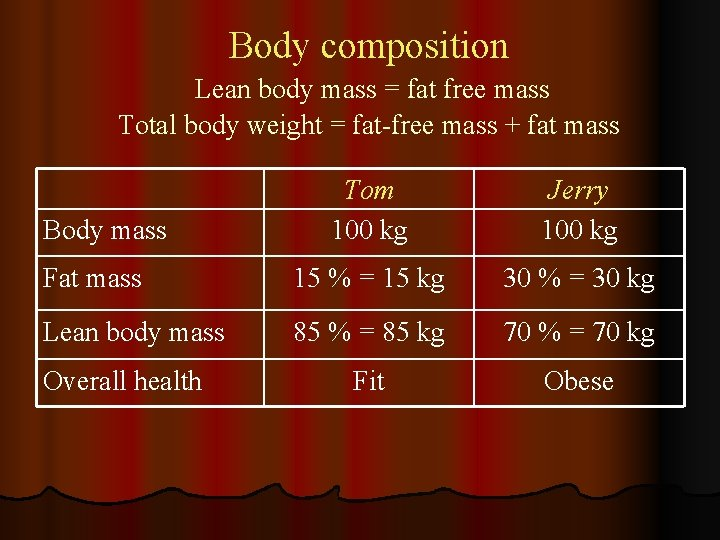Body composition Lean body mass = fat free mass Total body weight = fat-free mass + fat mass Tom 100 kg Jerry 100 kg Fat mass 15 % = 15 kg 30 % = 30 kg Lean body mass 85 % = 85 kg 70 % = 70 kg Fit Obese Body mass Overall health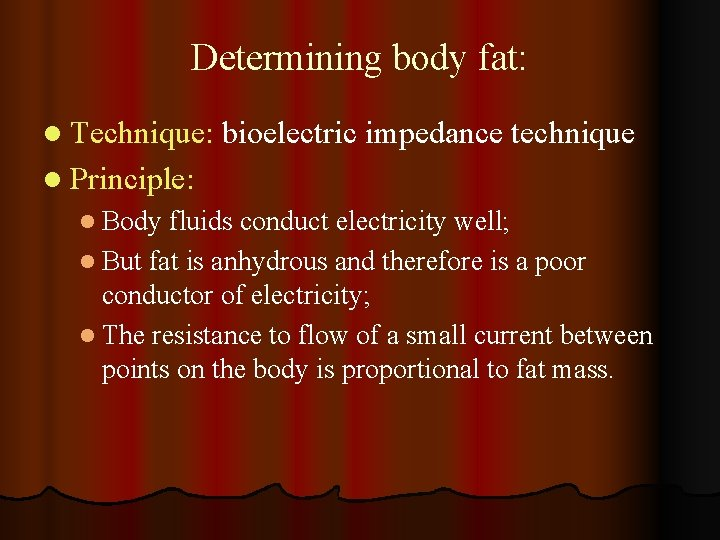Determining body fat: l Technique: bioelectric impedance technique l Principle: l Body fluids conduct electricity well; l But fat is anhydrous and therefore is a poor conductor of electricity; l The resistance to flow of a small current between points on the body is proportional to fat mass.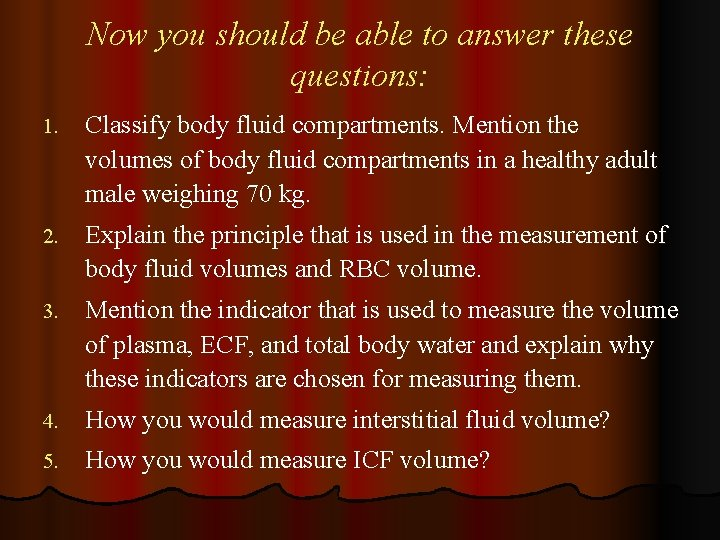Now you should be able to answer these questions: 1. Classify body fluid compartments. Mention the volumes of body fluid compartments in a healthy adult male weighing 70 kg. 2. Explain the principle that is used in the measurement of body fluid volumes and RBC volume. 3. Mention the indicator that is used to measure the volume of plasma, ECF, and total body water and explain why these indicators are chosen for measuring them. 4. How you would measure interstitial fluid volume? 5. How you would measure ICF volume?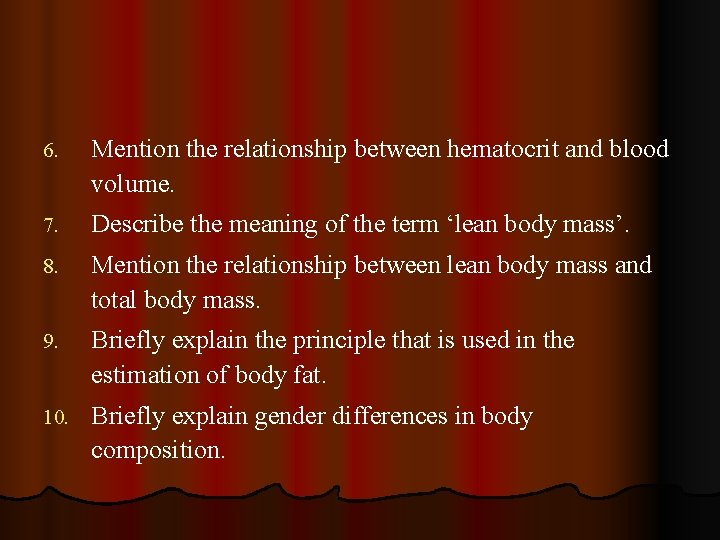6. Mention the relationship between hematocrit and blood volume. 7. Describe the meaning of the term ‘lean body mass’. 8. Mention the relationship between lean body mass and total body mass. 9. Briefly explain the principle that is used in the estimation of body fat. 10. Briefly explain gender differences in body composition.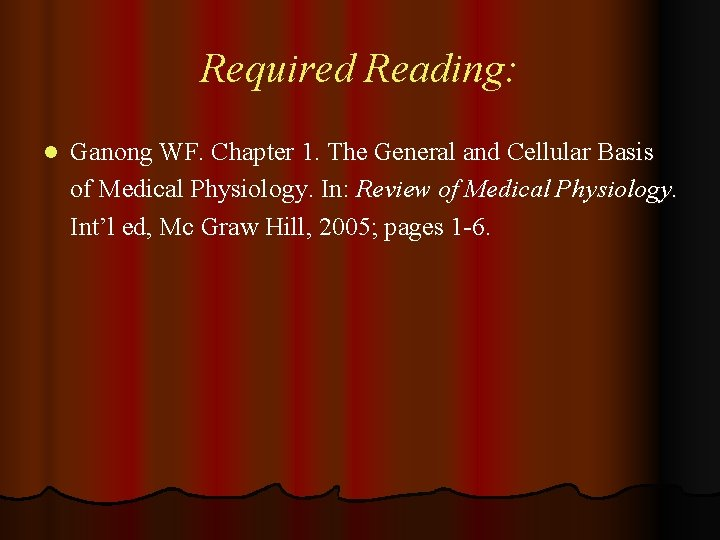Required Reading: l Ganong WF. Chapter 1. The General and Cellular Basis of Medical Physiology. In: Review of Medical Physiology. Int’l ed, Mc Graw Hill, 2005; pages 1 -6.